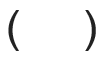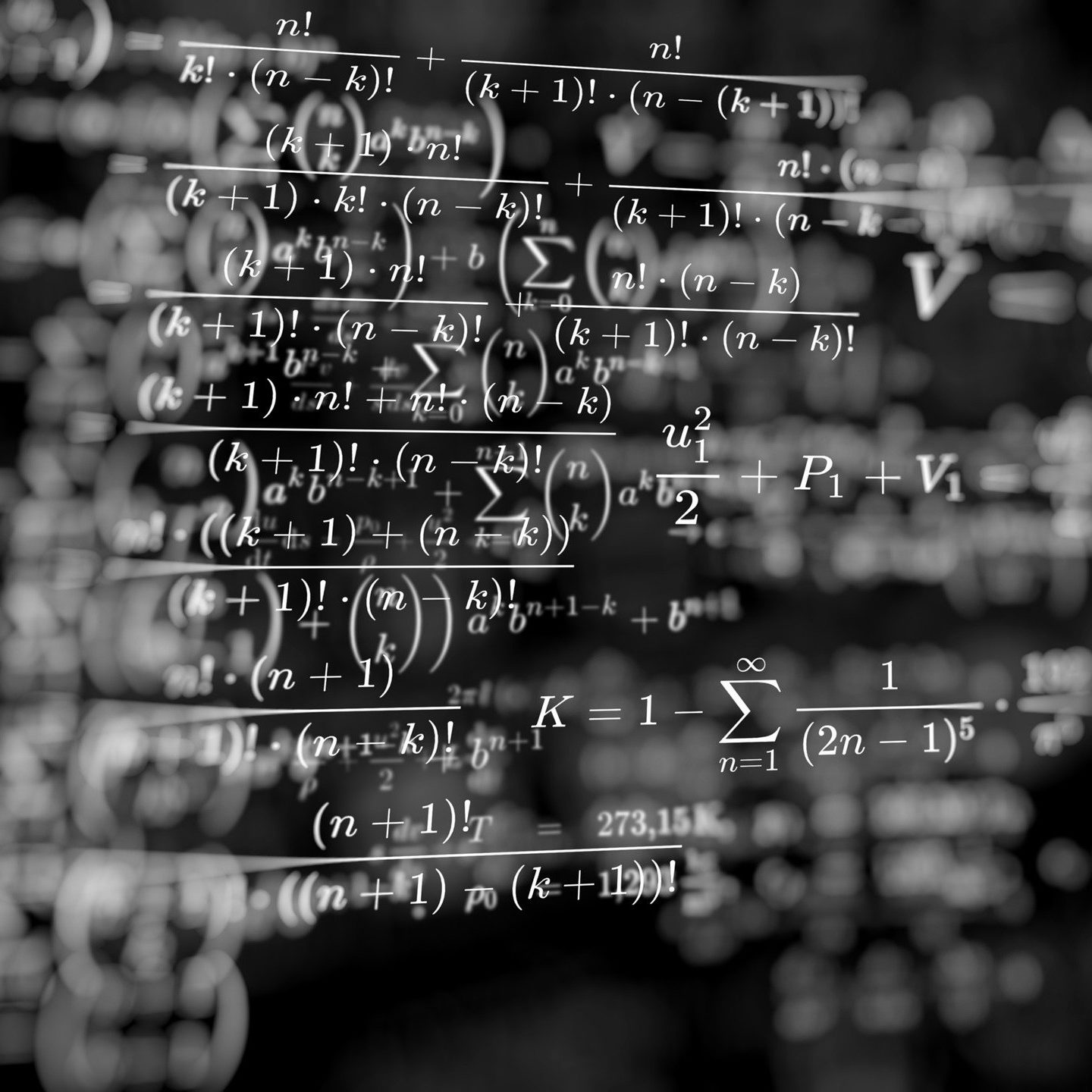Working with Equations

# 8 Order of Operations

Click play on the following audio player to listen along as you read this section.What does it mean when we talk about “order of operations” in math? Well the order of operations are a set of rules that need to be followed when dealing with math equations. Why do we need rules you ask? If there were no rules then you might end up with two different answers to the same math question.

Take a look at the following equation and calculate what you think the answer would be.

$\Large 5 + 4 \times 3 = ?$

$\Large \begin{array}{lrl}\text{Option A} & \text{Start with:} & 5 + 4 = 9 \\ & \text{Then:} & 9 \times 3 = 27 \end{array}$

$\Large \begin{array}{lrl} \text{Option B}& \text{Start with:} & 4 \times 3 = 12 \\ & \text{Then:} & 12 + 5 = 17 \end{array}$

The idea here is that you can’t have two answers for the same question. That just won’t work in math. One of the two answers must be correct and in both options the actual math (by that I mean if you plugged the numbers into a calculator) is correct. No mistakes were made. The issue is that the order of operations in one of the options is wrong.

And the correct answer is… Option B.

The next question then becomes “what are the rules to follow when dealing with equations?”

This is where the term “BEDMAS” comes into play.

# BedmasBedmas is an acronym used to identify the order of operations when dealing with math. When you have equations that use different operations such as addition, subtraction, multiplication, and division, BEDMAS sets out the order to do those calculations.

The example above shows us that having these guidelines will make sure that we don’t get the wrong answer when working through math problems.

BEDMAS

• Brackets
• Exponents
• Division
• Multiplication
• Subtraction

Bedmas tells us the order of operations when performing calculations within an equation. For example we solve exponents before we multiply, which in turn is done before we subtract.

One thing to note is that when working with division and multiplication or addition and subtraction, you will actually solve whichever comes first in the equation. So if multiplication comes before division, it is multiplication that is solved first. And if division comes before multiplication, then division is solved first. It is the same with addition and subtraction.

So when using Bedmas, it’s helpful to think of it like this:

• Brackets
• Exponents
• Division or Multiplication

We should all be familiar with division, multiplication, addition and subtraction at this point but what about dealing with brackets and exponents? What are they and how do they work?

Brackets are the first order of business in Bedmas and look like the following:Sometimes brackets can also be referred to as “parenthesis” and you may see the acronym PEDMAS used instead of BEDMAS. It means the same thing, and the symbol for parenthesis looks like the following:You can look at brackets as a mechanism that is used to group numbers or symbols together. The calculations within the brackets (or parenthesis) are then the first to be done.

For example:

$\Large \text{Z} = 4 \times 2 \times (5 \times 9)$

This situation would indicate the first calculation we perform is 5 × 9. Then we would work through the rest of the equation.

Exponents are the second order of operation in Bedmas. Have you ever looked at a math problem and seen the following?

$\Large \text{D} = 4 + 8 \times 5 - 3 + {9}^{3}$

Well the 93 is what we are talking about when dealing with exponents. Specifically we are dealing with the 3 portion.

Exponents tell you how many times you multiply a number by itself within an equation. In this case the 3 indicates that we multiply the 9 three times.

$\Large {9}^{3} = 9 \times 9 \times 9$

We can look at another example to see how exponents work.

$\Large {5}^{6} = 5 \times 5 \times 5 \times 5 \times 5 \times 5$

After brackets and exponents we move onto dividing, multiplying, adding and subtracting.

Let’s we go through a couple of examples to see if you get the idea.

Example

$\Large\text{A}=1+2\times 6\div3$

Although we won’t include this in the steps to answer the question, the first thing you might want to do is write down Bedmas so you can refer to it visually.

• Brackets
• Exponents
• Division
• Multiplication
• Subtraction

Step 1: There are no brackets in this equation and no exponents either. There is both division and multiplication. Because multiplication comes first, that is what we will solve first.

$\Large\text{A}=1+\mathbf{2\times6}\div3$

$\Large2\times6=12$

So now we have: $\Large\text{A}=1+12\div3$

Step 2: Now that we have solved the multiplication, we can solve the division.

$\Large\text{A}=1+\mathbf{12\div3}$

$\Large12\div3=4$

So now we have: $\Large\text{A}=1+4$

Step 3: We only have one more operation to go so we are nearing the end. Just add the one and four and we have our answer.

$\Large \begin{array}{c} \text{A} = 1 + 4 \\ \text{A} = 5 \end{array}$You can now see that if you didn’t follow the rules for order of operations, things could get a little fuzzy. There might be a few different answers you could come up with. Could you imagine writing a multiple-choice math exam where every answer seems to be right depending on how you did the math?

This is the importance of BEDMAS!

We’re probably at the point now where a few more examples are in order.

Example

Solve for Y.

$\Large \text{Y} = (24+36) \times 2 + {4}^{2}$

Step 1: Refer to BEDMAS. Work through the brackets first.

$\Large \begin{array}{c} \text{Y}=\mathbf{(24+36)} \times 2 + {4}^{2} \\ 24 + 36 = 60 \\ \text{Y} = 60 \times 2 +{4}^{2} \end{array}$

Step 2: Deal with the exponents next.

$\Large \begin{array}{c} \text{Y} = 60 \times 2 + \mathbf{{4}^{2}} \\ {4}^{2} = 4 \times 4 = 16 \\ \text{Y} = 60 \times 2 + 16 \end{array}$

Step 3: Work through the multiplying.

$\Large \begin{array}{c} \text{Y}= \mathbf{60 \times 2} + 16 \\ 60 \times 2 = 120 \\ \text{Y} = 120 + 16 \end{array}$

Step 4: The last step in this question is to do the addition.

$\Large \begin{array}{c} \text{Y} = 120 + 16 \\ \text{Y} = 136 \\ \text{Final Answer: Y} = 36 \end{array}$

Once you go through a few questions and learn to work things out using Bedmas, working through any math equation or formula becomes routine. After a while, you will just naturally know the steps to take.

Example

Solve for M.

$\Large \text{M} = {17}^{2} \times 24 + 13 + 7 \times (45 \div 5)$

Step 1: Work through the brackets first.

$\Large \begin{array}{c} \text{M} = {17}^{2} \times 24 + 13 + 7 \times \mathbf{(45 \div 5) }\\ 45 \div 5 = 9 \\ \text{M} = {17}^{2} \times 24 + 13 + 7 \times 9 \end{array}$

Step 2: Deal with the exponents next.

$\Large \begin{array}{c} \text{M} = \mathbf{{17}^{2}} \times 24 + 13 + 7 \times (45 \div 5) \\ {17}^{2} = 17 \times 17 = 289 \\ \text{M} = 289 \times 24 + 13 + 7 \times 9 \end{array}$

Step 3: Work through the multiplying.

$\Large \begin{array}{c} \text{M} = \mathbf{289 \times 24} + 13 + \mathbf{7 \times 9} \\ 289 \times 24 = 6936 \\ 7 \times 9 = 63 \\ \text{M} = 6939 + 13 + 63 \end{array}$

$\Large \begin{array}{c} \text{M} = 6939 + 13 + 63 \\ \text{M} = 7012 \\ \text{Final Answer: M} = 7012 \end{array}$

# Practice Questions

Try a couple practice questions for yourself and check the video answers to see how you did.

Question 1

Solve for D.

$\Large \text{D} = 5 + 6 \div 2 \times 7 + {4}^{3} \times (5+7)$

Question 2

Solve for R.

$\Large \text{R} = 17 + (6\times 3) + {5}^{2} \div 5 - 22$